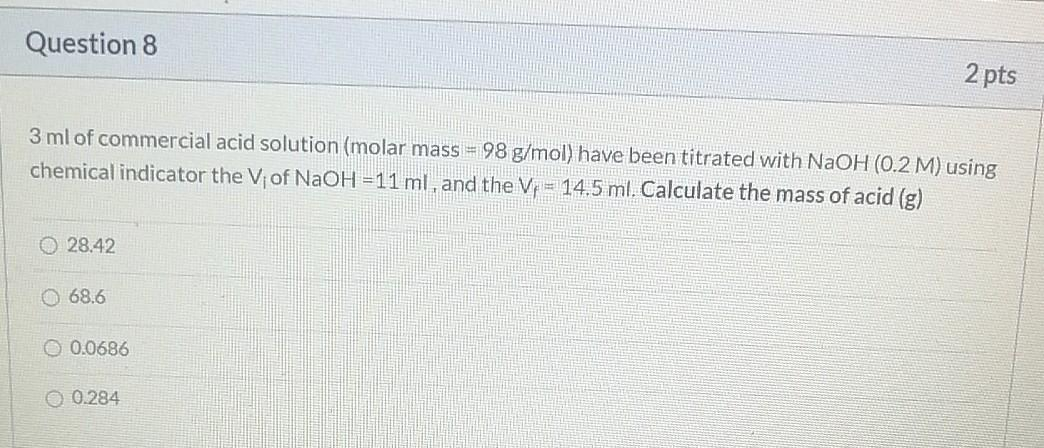### Create an Account

Home / Questions / 3 ml of commercial acid solution (molar mass =98 g/mol) have been titrated with NaOH (0.2...

# 3 ml of commercial acid solution (molar mass =98 g/mol) have been titrated with NaOH (0.2 M) using chemical indicator the Vi of NaOH = 11 ml, and the Vp= 14.5 ml. Calculate the mass of acid

3 ml of commercial acid solution (molar mass =98 g/mol) have been titrated with NaOH (0.2 M) using chemical indicator the Vi of NaOH = 11 ml, and the Vp= 14.5 ml. Calculate the mass of acid (g) 28.42 68.6 O 0.0686 0 0.284May 18 2021 View more View LessSubscribe To Get Solution